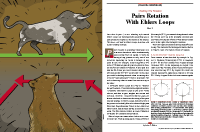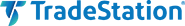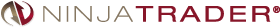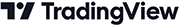# July 2022For this month’s Traders’ Tips, the focus is John Ehlers’ article in this issue, “Pairs Rotation With Ehlers Loops, Part 2.” Here, we present the July 2022 Traders’ Tips code with possible implementations in various software.

You can right-click on any chart to open it in a new tab or window and view it at it’s originally supplied size, often much larger than the version printed in the magazine.

The Traders’ Tips section is provided to help the reader implement a selected technique from an article in this issue or another recent issue. The entries here are contributed by software developers or programmers for software that is capable of customization.In part 1 of his article in the June 2022 issue (“Ehlers Loops”), John Ehlers uses a relationship of price and volume to determine if any predictive value can be obtained. His technique, called Ehlers Loops, aid in visualizing the performance of one datastream against another. In part 2 appearing in this issue (“Pairs Rotation With Ehlers Loops”), the author demonstrates Ehlers Loops in action with a pairs trading example. This pairs rotation strategy is aimed at minimizing drawdown while simultaneously maximizing return on capital.

Note that the code assumes that the export directory “C:\Temp” exists and that the indicator is applied to “daily and above” bar timeframes. To plot Ehlers Loops in Excel, after opening the file, highlight the second and third columns (B and C) for the desired date range and click on insert and choose “scatter plot with smooth lines and indicators.”

```// TASC JUL 2022
// Ehlers Loops Pairs Rotation Chart
// (C) 2005-2022 John F. Ehlers

inputs:
LPPeriod( 20 ),
HPPeriod( 125 );

variables:
HP1( 0 ),
HP2( 0 ),
hpa1( 0 ),
hpb1( 0 ),
hpc1( 0 ),
hpc2( 0 ),
hpc3( 0 ),
ssa1( 0 ),
ssb1( 0 ),
ssc1( 0 ),
ssc2( 0 ),
ssc3( 0 ),
Price1( 0 ),
Price1MS( 0 ),
Price1RMS( 0 ),
Price2( 0 ),
Price2MS( 0 ),
Price2RMS( 0 );

if CurrentBar = 1 then
begin
hpa1 = expvalue(-1.414*3.14159 / HPPeriod);
hpb1 = 2*hpa1*Cosine(1.414*180 / HPPeriod);
hpc2 = hpb1;
hpc3 = -hpa1*hpa1;
hpc1 = (1 + hpc2 - hpc3) / 4;
ssa1 = expvalue(-1.414*3.14159 / LPPeriod);
ssb1 = 2*ssa1*Cosine(1.414*180 / LPPeriod);
ssc2 = ssb1;
ssc3 = -ssa1*ssa1;
ssc1 = 1 - ssc2 - ssc3;
End;

// Normalized Roofing Filter for Data1
// (horizontal plot)
// 2 Pole Butterworth Highpass Filter
HP1 = hpc1*(Close of Data1 - 2*Close of Data1 + Close of
Data1) + hpc2*HP1 + hpc3*HP1;

if CurrentBar < 3 then
HP1 = 0;

// Smooth with a Super Smoother Filter
Price1 = ssc1*(HP1 + HP1) / 2 + ssc2*Price1
+ ssc3*Price1;

if CurrentBar < 3 then Price1 = 0;
// Scale Price in terms of Standard Deviations

if CurrentBar = 1 then
Price1MS = Price1 * Price1
else
Price1MS = .0242*Price1*Price1
+ .9758*Price1MS;

if Price1MS <> 0 then
Price1RMS = Price1 / SquareRoot(Price1MS);

// Normalized Roofing Filter for Data2
// (horizontal plot)
// 2 Pole Butterworth Highpass Filter
HP2 = hpc1*(Close of Data2 - 2*Close of Data2
+ Close of Data2) + hpc2*HP2 + hpc3*HP2;

if CurrentBar < 3 then
HP2 = 0;

// Smooth with a Super Smoother Filter
Price2 = ssc1*(HP2 + HP2) / 2 + ssc2*Price2 +
ssc3*Price2;

if CurrentBar < 3 then
Price2 = 0;

// Scale Price in terms of Standard Deviations
if CurrentBar = 1 then
Price2MS = Price2*Price2
else
Price2MS = .0242*Price2*Price2 + .9758*Price2MS;

if Price2MS <> 0 then
Price2RMS = Price2 / SquareRoot(Price2MS);

// Conventional Plots
Plot1( Price1RMS, "Price1 RMS" );
Plot2( 0, "Zero Line" );
Plot3( Price2RMS, "Price2 RMS" );
```

A sample chart is shown in Figure 1.FIGURE 1: TRADESTATION. Here, the Ehlers Loops pairs rotation indicator is applied in the subgraph on a TradeStation daily chart of Raytheon Technologies (RTX) and the S&P 500 ETF SPY.

—Chris Imhof

BACK TO LIST### Wealth-Lab.com: July 2022

Wealth-Lab 8 makes it easy to display various custom plots such as line or bar charts, pie graphs, and scatter plots with just a few lines of code. The code below accomplishes this with an open-source plotting library for .NET called ScottPlot.

With a little tweak, the same C# code that we presented last month in the June 2022 Traders’ Tips section based on part 1 of John Ehlers’ article, “Ehlers Loops,” can be used to create Ehlers Loops applied to pairs, which is the technique he demonstrates in part 2 of his article in this issue, “Pairs Rotation With Ehlers Loops.”

```using WealthLab.Backtest;
using System;
using WealthLab.Core;
using WealthLab.Indicators;
using System.Drawing;
using System.Collections.Generic;
using ScottPlot;

namespace WealthScript1
{
public class TASC202207PairsRotationWithEhlersLoops : UserStrategyBase
{
/* create indicators and other objects here, this is executed prior to the main trading loop */
public override void Initialize(BarHistory bars)
{
spy = GetHistory(bars, "SPY");
PlotBarHistory(spy, "SPYPane", WLColor.Silver);

int LPPeriod = 20, HPPeriod = 125;
double Deg2Rad = Math.PI / 180.0;
List<double> lstDates = new List<double>();
List<double> lstValuesP = new List<double>();
List<double> lstValuesP2 = new List<double>();

hpa1 = Math.Exp(-1.414 * Math.PI / HPPeriod);
hpb1 = 2.0 * hpa1 * Math.Cos((1.414 * 180d / HPPeriod) * Deg2Rad);
hpc2 = hpb1;
hpc3 = -hpa1 * hpa1;
hpc1 = (1 + hpc2 - hpc3) / 4;
ssa1 = Math.Exp(-1.414 * Math.PI / LPPeriod);
ssb1 = 2.0 * ssa1 * Math.Cos((1.414 * 180d / LPPeriod) * Deg2Rad);
ssc2 = ssb1;
ssc3 = -ssa1 * ssa1;
ssc1 = 1 - ssc2 - ssc3;

/* 2 Pole Butterworth Highpass Filter */
TimeSeries HP1 = new TimeSeries(bars.DateTimes, 0);
TimeSeries HP2 = new TimeSeries(spy.DateTimes, 0);
for (int i = 2; i < bars.DateTimes.Count; i++)
{
HP1[i] = hpc1 * (bars.Close[i] - 2 * bars.Close[i - 1] + bars.Close[i - 2]) + hpc2 * HP1[i - 1] + hpc3 * HP1[i - 2];
HP2[i] = hpc1 * (spy.Close[i] - 2 * spy.Close[i - 1] + spy.Close[i - 2]) + hpc2 * HP2[i - 1] + hpc3 * HP2[i - 2];
}

/* Smooth with a Super Smoother Filter */
TimeSeries Price = new TimeSeries(bars.DateTimes, 0);
TimeSeries Price2 = new TimeSeries(spy.DateTimes, 0);
for (int i = 2; i < bars.DateTimes.Count; i++)
{
Price[i] = ssc1 *(HP1[i] + HP1[i - 1]) / 2 + ssc2 *Price[i - 1] + ssc3 *Price[i - 2];
Price2[i] = ssc1 *(HP2[i] + HP2[i - 1]) / 2 + ssc2 *Price2[i - 1] + ssc3 *Price2[i - 2];
}

/* Scale Price in terms of Standard Deviations */
TimeSeries PriceMS = new TimeSeries(bars.DateTimes, 0);
TimeSeries PriceRMS = new TimeSeries(bars.DateTimes, 0);
TimeSeries Price2MS = new TimeSeries(spy.DateTimes, 0);
TimeSeries Price2RMS = new TimeSeries(spy.DateTimes, 0);
for (int i = 0; i < bars.DateTimes.Count; i++)
{
if (i < 2)
{
PriceMS[i] = Math.Pow(Price[i], 2);
Price2MS[i] = Math.Pow(Price2[i], 2);
}
else
{
PriceMS[i] = 0.0242 * Price[i] * Price[i] + 0.9758 * PriceMS[i - 1];
Price2MS[i] = 0.0242 * Price2[i] * Price2[i] + 0.9758 * Price2MS[i - 1];
}

if(PriceMS[i] != 0)
PriceRMS[i] = Price[i] / Math.Sqrt(PriceMS[i]);
if (Price2MS[i] != 0)
Price2RMS[i] = Price2[i] / Math.Sqrt(Price2MS[i]);

}

Bitmap bmp = null;
Plot plt = new ScottPlot.Plot(800, 600);
plt.Style(figureBackground: Color.FromArgb(51,53,54), dataBackground: Color.FromArgb(51,53,54), titleLabel: Color.White, grid: Color.White, axisLabel: Color.FromArgb(51,53,54));

plt.Title("Ehlers Loops");
plt.YLabel(bars.Symbol);
plt.XLabel(spy.Symbol);

plt.PlotScatter(lstValuesP2.ToArray(), lstValuesP.ToArray(), Color.Gold);
bmp = plt.GetBitmap();
DrawImageAt(bmp, 40, 20);
}

public override void Execute(BarHistory bars, int idx)
{
}

double hpa1, hpb1, hpc2, hpc3, hpc1, ssa1, ssb1, ssc2, ssc3, ssc1;
BarHistory spy;
}
}
```

Figure 2 shows an example chart of Ehlers Loops superimposed on a chart of RTX vs. SPY in Wealth-Lab 8.FIGURE 2: WEALTH-LAB. Ehlers Loops are superimposed on a chart of RTX vs. SPY in Wealth-Lab 8.

—Gene Geren (Eugene)
Wealth-Lab team
www.wealth-lab.com

BACK TO LISTThe Ehlers Loops pairs rotation indicator, as discussed in John Ehlers’ article in this issue, “Pairs Rotation With Ehlers Loops,” is available for download from the following links for NinjaTrader 8 and for NinjaTrader 7:

You can review the indicator’s source code in NinjaTrader 8 by selecting the menu New → NinjaScript Editor → Indicators from within the control center window and selecting the “Ehlers Loops Pairs Rotation” file. You can review the indicator’s source code in NinjaTrader 7 by selecting the menu Tools → Edit NinjaScript → Indicator from within the control center window and selecting the “Ehlers Loops Pairs Rotation” file.

NinjaScript uses compiled DLLs that run native, not interpreted, which provides you with the highest performance possible.

A sample chart displaying the indicator is shown in Figure 3.FIGURE 3: NINJATRADER. This shows an example of an Ehlers Loop pairs rotation of RTX versus SPY for four months in 2021. The loop plot starts on August 2nd.

—Kate Windheuser

BACK TO LISTThe John Ehlers’ Ehlers Loops pairs rotation values can be easily computed in NeuroShell Trader using NeuroShell Trader’s ability to call external dynamic linked libraries. Dynamic linked libraries can be written in C, C++, or Power Basic.

After moving the code given in John Ehlers’ article in this issue (“Pairs Rotation With Ehlers Loops”) to your preferred compiler and creating a DLL, you can insert the resulting indicator as follows:

1. Select “New Indicator” from the insert menu.
2. Choose the External Program & Library Calls category.
3. Select the appropriate External DLL Call indicator.
4. Setup the parameters to match your DLL.
5. Select the finished button.

To calculate the pair rotation loops, load a chart for the first security, insert the second security’s price onto the chart using “insert other instrument data,” then apply the Ehlers Loop to the price of each pair. Then simply use the NeuroShell Trader export feature to export the loop values to an Excel spreadsheet as described in Ehlers’ article.

An example chart in Figure 4 shows the calculated Ehlers Loops values for RTX vs. SPY.FIGURE 4: NEUROSHELL TRADER. This NeuroShell Trader chart shows the calculated Ehlers Loops values for RTX vs. SPY.

Users of NeuroShell Trader can go to the Stocks & Commodities section of the NeuroShell Trader free technical support website to download a copy of this or any previous Traders’ Tips.

—Ward Systems Group, Inc.
sales@wardsystems.com
www.neuroshell.com

BACK TO LISTThe importable TradersStudio files based on John Ehlers’ article in this issue, “Pairs Rotation With Ehlers Loops,” can be obtained on request via email to info@TradersEdgeSystems.com. The code is also shown below.

• Function EHLERS_LOOPS2: Computes normalized price data for two pairs of securities
• Indicator plot EHLERS_LOOPS2_SYS: A system code file for a session that exports data to the terminal. Once the session is run, the data can be copied from the terminal window to Excel and then plotted on a scatter plot.
```'Ehlers Loops2, TASC July 2022
'Author: John F. Ehlers
'Coded by: Richard Denning, 5/18/2022

'Ehlers Loops Pairs Rotation Chart
'(C) 2005-2022 John F. Ehlers

Function EHLERS_LOOPS2(LPPeriod, HPPeriod, IndPrice2 As BarArray, ByRef Price1RMS)

Dim HP1 As BarArray
Dim HP2 As BarArray
Dim hpa1 As BarArray
Dim hpb1 As BarArray
Dim hpc1 As BarArray
Dim hpc2 As BarArray
Dim hpc3 As BarArray
Dim ssa1 As BarArray
Dim ssb1 As BarArray
Dim ssc1 As BarArray
Dim ssc2 As BarArray
Dim ssc3 As BarArray
Dim Price1 As BarArray
Dim Price1MS As BarArray
'Dim Price1RMS As BarArray
Dim Price2 As BarArray
Dim Price2MS As BarArray
Dim Price2RMS As BarArray

'LPPeriod = 20
'HPPeriod = 125

If BarNumber = FirstBar Then
hpa1 = TStation_ExpValue(-1.414*3.14159 / HPPeriod)
hpb1 = 2*hpa1*TStation_Cosine(1.414*180 / HPPeriod)
hpc2 = hpb1
hpc3 = -hpa1*hpa1
hpc1 = (1 + hpc2 - hpc3) / 4
ssa1 = TStation_ExpValue(-1.414*3.14159 / LPPeriod)
ssb1 = 2*ssa1*TStation_Cosine(1.414*180 / LPPeriod)
ssc2 = ssb1
ssc3 = -ssa1*ssa1
ssc1 = 1 - ssc2 - ssc3
End If
HP1 = hpc1*(C - 2*C+ C) + hpc2*HP1 + hpc3*HP1

Price1 = ssc1*(HP1 + HP1) / 2 + ssc2*Price1 + ssc3*Price1

If BarNumber = FirstBar Then
Price1MS = Price1*Price1
Else
Price1MS = 0.0242*Price1*Price1 + 0.9758*Price1MS
End If
If Price1MS <> 0 Then
Price1RMS = Price1 / Sqr(Price1MS)
End If
HP2 = hpc1*(IndPrice2 - 2*IndPrice2 + IndPrice2) + hpc2*HP2 + hpc3*HP2

Price2 = ssc1*(HP2 + HP2) / 2 + ssc2*Price2 + ssc3*Price2

If BarNumber = FirstBar Then
Price2MS = Price2*Price2
Else
Price2MS = 0.0242*Price2*Price2 + 0.9758*Price2MS
End If
If Price2MS <> 0 Then
Price2RMS = Price2 / Sqr(Price2MS)
End If
EHLERS_LOOPS2 = Price2RMS

End Function
'---------------------------------------------------------------------------------------
'A System Code File to Export Data to Terminal Window
'Which Can Then Be pasted Into Excel For Plotting on a Scatter Graph
'Use This Code To Setup a Session

Sub EHLERS_LOOPS2_SYS(LPPeriod, HPPeriod)
'LLPeriod = 20, HPPeriod = 125

Dim Price1RMS As BarArray
Dim Price2RMS As BarArray
Dim VolRMS As BarArray
Dim IndPrice2 As BarArray
IndPrice2 = C of independent1
Price2RMS = EHLERS_LOOPS2(LPPeriod, HPPeriod, IndPrice2, Price1RMS)
Print FormatDateTime(Date)," Price1RMS ",Round(Price1RMS,2)," Price2RMS ",Round(Price2RMS,2)
End Sub
'--------------------------------------------------------------------------------------------
```

Figure 5 shows a scatter plot using data from the terminal window.FIGURE 5: TRADERSSTUDIO. Ehlers Loops pairs are shown on a scatter plot of eBay (EBAY) vs. Amazon (AMZN) for a one-year period ending 7/11/2014.

—Richard Denning

BACK TO LIST### The Zorro Project: July 2022

In his article last month, John Ehlers presented a new way to display price over volume in a loop plot. This month, in his article in this issue, “Pairs Rotation With Ehlers Loops,” he uses the same method to compare a stock with an index for pairs rotation.

The C code for the roofing filter that smooths and scales the data is the same:

```var Roofing(var *Data,int HPeriod,int LPeriod)
{
var f = 1.414*PI/HPeriod;
var hpa1 = exp(-f);
var hpc2 = 2*hpa1*cos(f/2);
var hpc3 = -hpa1*hpa1;
var hpc1 = (1 + hpc2 - hpc3) / 4;
f = 1.414*PI/LPeriod;
var ssa1 = exp(-f);
var ssc2 = 2*ssa1*cos(f/2);
var ssc3 = -ssa1*ssa1;
var ssc1 = 1 - ssc2 - ssc3;

vars HP = series(0,3);
HP = hpc1*(Data - 2*Data + Data) + hpc2*HP + hpc3*HP;
vars SS = series(HP,3);
SS = ssc1*(HP + HP)/2 + ssc2*SS + ssc3*SS;
var Scaled = EMA(SS*SS,.0242);
return SS/sqrt(Scaled);
}
```

We’re now applying that filter to the price series of the two instruments and display the result in a scatter plot. The code for replicating the RTX/SPY chart from Ehlers’ article in this issue follows:

```function run()
{
BarPeriod = 1440;
LookBack = 300;
StartDate = 20210801;
EndDate = 20211130;

asset("RTX");
var Y = Roofing(seriesC(),125,20);
asset("SPY");
var X = Roofing(seriesC(),125,20);

if(is(LOOKBACK)) return;
plotGraph("Loop",X,Y,DOT|GRAPH,BLUE);
plotGraph("Loops",X,Y,SPLINE|GRAPH,TRANSP|GREY);
if(is(EXITRUN))
plotGraph("Last",X,Y,SQUARE|GRAPH,RED);
}
```

The plotGraph functions mark each coordinate with a blue dot and connect the dots with spline lines. The last day is marked with a red square. The resulting chart is shown in Figure 6.FIGURE 6: ZORRO PROJECT. The roofing filter is applied to the price series of two instruments and the results are displayed in a scatter plot. The last day is marked with a red square.

I found that Ehlers’ roofing filter is sensitive to the lookback period. It needs some time to “swing in.” A too-short lookback period, like Zorro’s default 80 bars, produces a visibly different loop.

Ehlers proposes to switch between stock and index depending on the loop rotation and angle quadrant. This was intended for discretionary trading, but can theoretically also be automated with a script.

The roofing indicator and the Ehlers Loop script can be downloaded from the 2022 script repository on https://financial-hacker.com. The Zorro software can be downloaded from https://zorro-project.com.

—Petra Volkova
The Zorro Project by oP group Germany
https://zorro-project.com

BACK TO LIST

### Microsoft Excel: July 2022

In his article in this issue, “Pairs Rotation With Ehlers Loops,” John Ehlers provides an example of using Ehlers Loops for pairs trading. There is a lot of information to be gleaned when working with Ehlers Loops in a pairs rotation.

Let’s start with Figure 7, which replicates Ehlers’ example from his article in this issue. From there we will drill down a bit.FIGURE 7: EXCEL. This chart replicates Figure 3 from John Ehlers’ article in this issue.

In Figure 8 we have the RTX component pricing and RMS charts. The peak and decline of the RMS plot near the center looks to be anticipating the price action. (The cursors in Figures 7 and 8 are on the same bar date.)FIGURE 8: EXCEL. Here, the price chart and root mean square (RMS) chart for Raytheon Technologies (RTX) is shown, with the cursor positioned to match Figure 7 above.

Figure 9 displays the result of playing with the HP and LP settings. In this instance, the RMS plots are noisier and seem to foreshadow the eventual price action to a greater degree than the settings used in Figure 8.FIGURE 9: EXCEL. Shown here the loop components for RTX and SPY.

Figures 10, 11, and 12 give a larger detail view of the pairs comparison with the 80,10 HP-LP period settings in Figure 9.FIGURE 10: EXCEL. This shows an example of what happens to the root mean square (RMS) values when you experiment with the HP and LP periods.FIGURE 11: EXCEL. This shows another example of what happens to the root mean square (RMS) values when you experiment with the HP and LP periods. Compare to Figure 9 above, which used 80,10 HP-LP period settings.FIGURE 12: EXCEL. Shown here is a loop chart for the 80, 10 HP and LP settings.

When experimenting with the spreadsheet I am providing here, please be sure to use the cursor slider to get a feel for what price points generated any particular point on the Ehlers Loop and how the direction of the move along the loop to the next point works for you as a visual predictor of future price action. Be sure to try other HP and LP period values.

• Right-click on the Excel file link, then
• Select “save as” (or “save target as”) to place a copy of the spreadsheet file on your hard drive.

—Ron McAllister
Excel and VBA programmer
rpmac_xltt@sprynet.com

BACK TO LISTHere is the Pine Script code for TradingView that implementing the Ehlers Loops described in this issue’s article “Pairs Rotation With Ehlers Loops” by John F. Ehlers.

```//  TASC Issue: July 2022 - Vol. 40, Issue 8
//     Article: Pairs Rotation With Ehlers Loops
//              Part 2 - Charting The Rotation
//  Article By: John F. Ehlers
//    Language: TradingView's Pine Script v5
// Provided By: PineCoders, for tradingview.com

//@version=5
indicator("TASC 2022.07 Pairs Rotation With Ehlers Loops",
"PRWEL", max_lines_count=300, max_labels_count=300)

//== 2 Pole Butterworth Highpass Filter ==//
butterworthHP(float Series, float Period) =>
var float ALPHA =  math.pi * math.sqrt(2.0) / Period
var float BETA  =  math.exp(-ALPHA )
var float COEF2 = -math.pow(BETA, 2)
var float COEF1 =  math.cos( ALPHA ) * 2.0 * BETA
var float COEF0 =  (1.0 + COEF1 - COEF2) * 0.25
float tmp    = nz(Series,  Series)
float whiten =    Series + nz(Series, tmp) - 2.0 * tmp
float smooth = na, smooth := COEF0 *     whiten     +
COEF1 *  nz(smooth) +
COEF2 *  nz(smooth)

//===== 2 Pole Super Smoother Filter =====//
superSmoother(float Series, float Period) =>
var float ALPHA =  math.pi * math.sqrt(2.0) / Period
var float BETA  =  math.exp(-ALPHA )
var float COEF2 = -math.pow(BETA, 2)
var float COEF1 =  math.cos( ALPHA ) * 2.0 * BETA
var float COEF0 =  1.0 - COEF1 - COEF2
float sma2   = math.avg(Series, nz(Series, Series))
float smooth = na, smooth := COEF0 *      sma2      +
COEF1 *  nz(smooth) +
COEF2 *  nz(smooth)

//===== Faster Root Mean Square =====//
fastRMS(float Series, float Period) =>
if Period < 1
runtime.error("Err: fastRMS(Period=) is less than 1")
var float COEF0 = 2.0 / (Period + 1)
var float COEF1 = 1.0 -  COEF0
float pow = math.pow(Series, 2)
float ema = na, ema := COEF0 *    pow +
COEF1 * nz(ema, pow)
nz(Series / math.sqrt(ema))

//===== RMS Scaled Bandpass Filter =====//
scaledFilt(int periodHP, int periodLP, int periodRMS) =>
float HP       = butterworthHP(close, periodHP )
float BPF      = superSmoother(   HP, periodLP )
float priceRMS =       fastRMS(  BPF, periodRMS)

//===== Draws a Vertcal Line Segment =====/
vline(int X, color Color) =>
var zero = line.new(X, -3.0, X, 3.0, color=Color,
style=line.style_dotted,  width=1)
line.set_x1(zero, X+1)
line.set_x2(zero, X)

//======= Draws Various Deviation Boxes =======//
drawDeviationBox(float Width, int Offset, float Height,
int Deviations, color Color) =>
if barstate.islast
var Box = box.new(
bar_index, Height * 0.5, bar_index, Height * -0.5,
border_color=Color, border_style=line.style_dotted,
bgcolor=color.new(Color, 96), text_size=size.small,
text=str.tostring(Deviations)   +  ' deviations',
text_color=Color,  text_valign=text.align_bottom)
width = int(Width)
box.set_left( Box, int(bar_index - width) + Offset)
box.set_right(Box, int(bar_index + width) + Offset)

//===== Draws the Ehlers Loops =====/
drawLoop(float xPosition, float yPosition, color  Color,
int  Segments,   int  BarScale,   int Offset) =>
var aSegments = array.new<line> (Segments  )
var aNodes    = array.new<label>(Segments+1)
if barstate.islast
for i=0 to Segments
float Y2 =     yPosition[  i]
int   X2 = int(xPosition[  i] * BarScale)
int   X1 = int(xPosition[1+i] * BarScale)
float Y1 =     yPosition[1+i]
X2 := math.min(500, X2 + Offset) + bar_index
X1 := math.min(500, X1 + Offset) + bar_index
if i < Segments
width = int(4 *(Segments - i) / Segments + 1)
line.delete(array.get(aSegments, i))
segment= line.new(X1, Y1, X2, Y2, color=Color,
style=line.style_solid,  width=width)
array.set( aSegments, i, segment)
nodeSize = i==0 ? size.large : size.normal
nodeChar = i==0 ? 'â¦¿' : i==Segments ? 'ðŸ—™' : 'â¯'
label.delete(array.get(aNodes, i))
node = label.new(X2, Y2, nodeChar, size=nodeSize,
color=#00000000, textcolor=Color,
style=label.style_label_center)
array.set(aNodes, i, node)

pairedSym = input.symbol('SP:SPX','Paired Symbol')
periodLP  = input.int( 20,  "Low-Pass Period", minval= 7)
periodHP  = input.int(125, "High-Pass Period", minval=20)
periodRMS = input.int( 80,       "RMS Period",   step=10)
colorLoop = input.color(#FF0000, 'Loop Color')
barScale  = input.int( 40,       'Bar Scale:',   step= 5)
offset    = input.int(125, 'X Axis Position:',   step= 5)
segments  = input.int( 50,   'Loop Segments:', minval=10)

expression = scaledFilt(periodHP, periodLP, periodRMS)

var  CHART_TICKER = ticker.new(syminfo.prefix, syminfo.ticker)
var PAIRED_SYMBOL = ticker.new(syminfo.prefix(pairedSym),
syminfo.ticker(pairedSym))
PriceRMS_1 = request.security( CHART_TICKER, timeframe.period,
expression, ignore_invalid_symbol=true)
PriceRMS_2 = request.security(PAIRED_SYMBOL, timeframe.period,
expression, ignore_invalid_symbol=true)

plot(PriceRMS_1,  'Chart Symbol', color=#FF5555)
plot(PriceRMS_2, 'Paired Symbol', color=#5555FF)

hline(    0.0, "Zero", #808000FF, hline.style_dotted)
vline(math.min(500, offset) + bar_index, #808000FF)
drawDeviationBox(3.0 * barScale, offset, 6.0, 3, #FF0000)
drawDeviationBox(2.0 * barScale, offset, 4.0, 2, #FF6600)
drawDeviationBox(      barScale, offset, 2.0, 1, #FFCC00)

drawLoop(PriceRMS_2, PriceRMS_1, colorLoop,
segments,   barScale,    offset)

```

An example chart is shown in Figure 13.